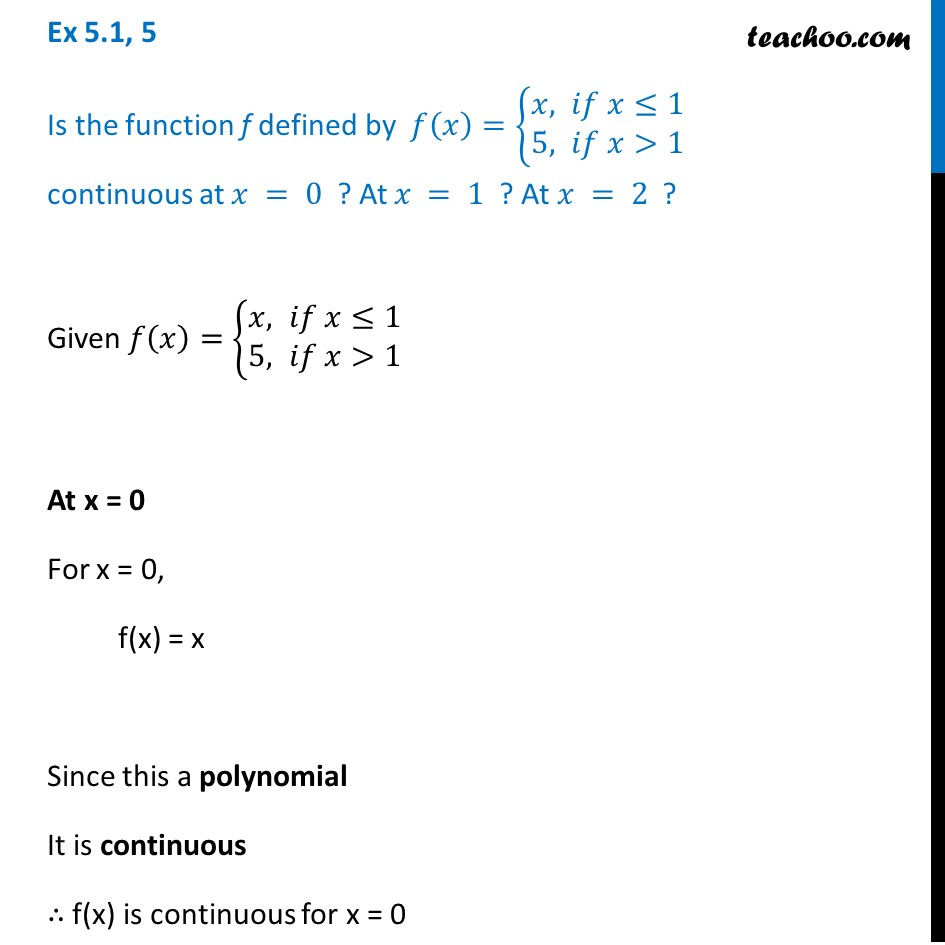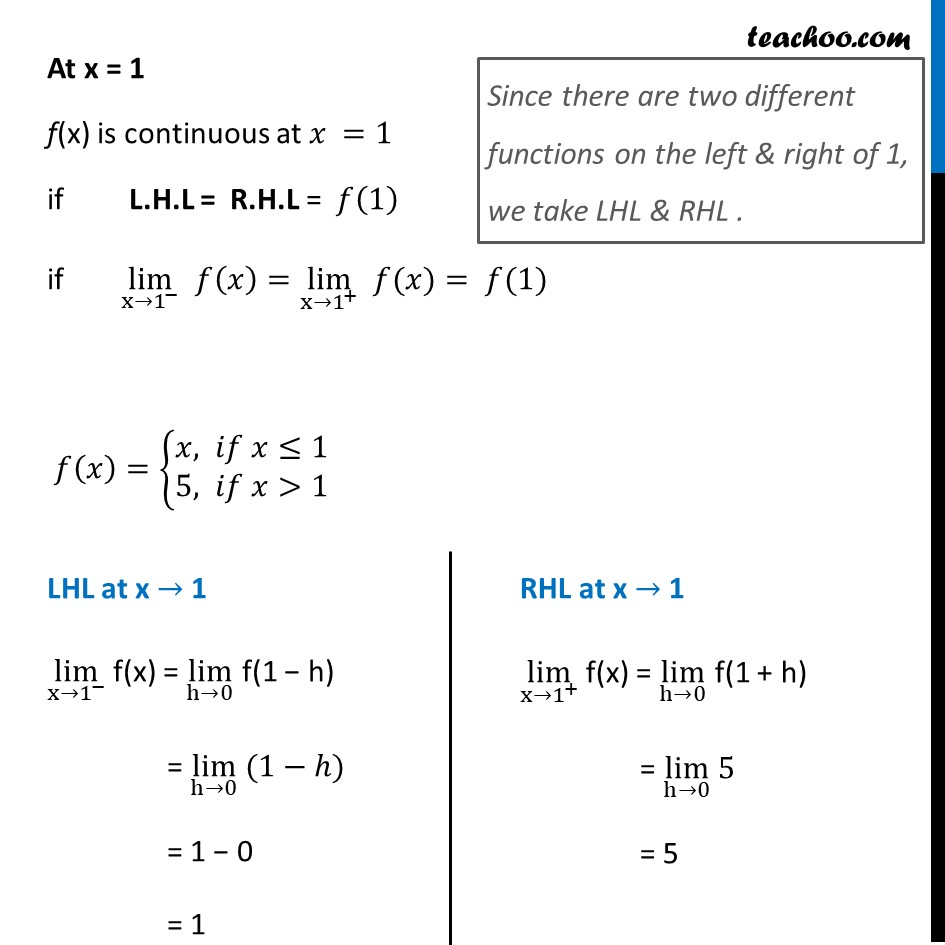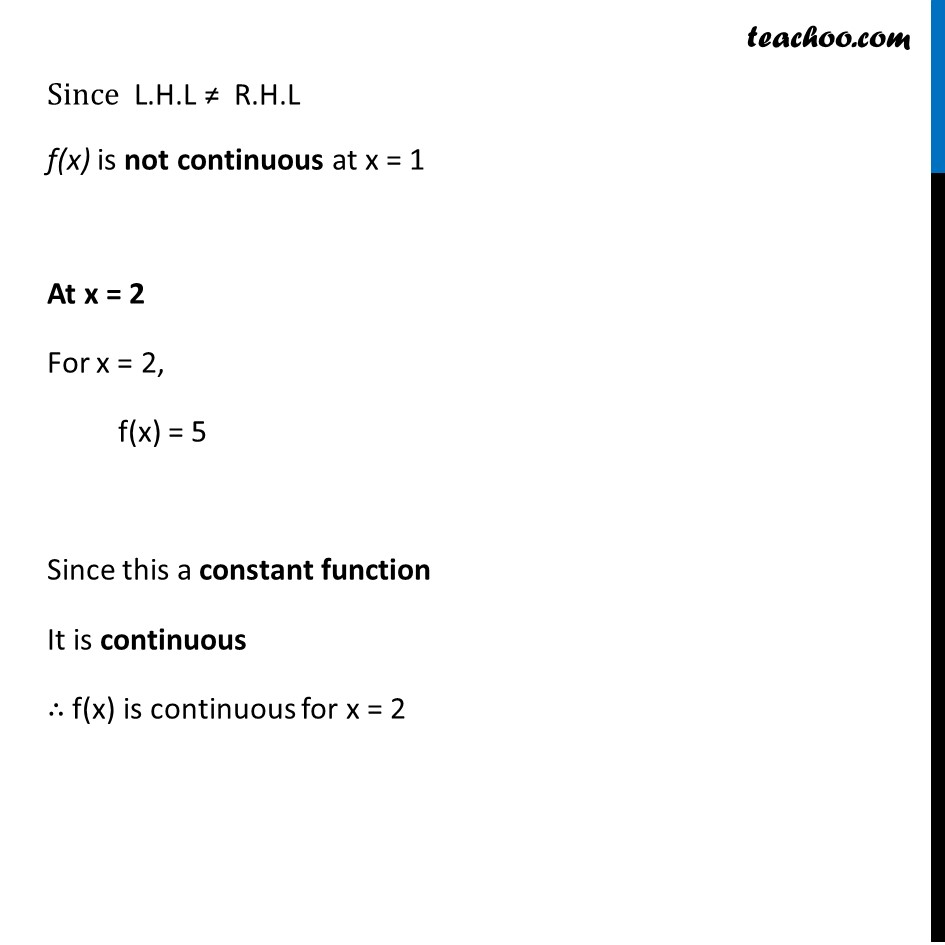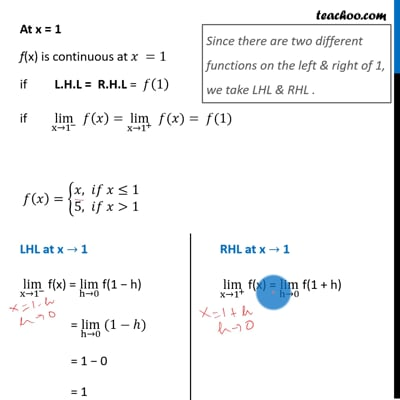Ex 5.1

Chapter 5 Class 12 Continuity and Differentiability
Serial order wiseThis video is only available for Teachoo black users

Introducing your new favourite teacher - Teachoo Black, at only ₹83 per month

### Transcript

Ex 5.1, 5 Is the function f defined by 𝑓(𝑥)={█(𝑥, 𝑖𝑓 𝑥≤1@&5, 𝑖𝑓 𝑥>1)┤ continuous at 𝑥 = 0 ? At 𝑥 = 1 ? At 𝑥 = 2 ? Given 𝑓(𝑥)={█(𝑥, 𝑖𝑓 𝑥≤1@&5, 𝑖𝑓 𝑥>1)┤ At x = 0 For x = 0, f(x) = x Since this a polynomial It is continuous ∴ f(x) is continuous for x = 0 At x = 1 f(x) is continuous at 𝑥 =1 if L.H.L = R.H.L = 𝑓(1) if lim┬(x→1^− ) 𝑓(𝑥)=lim┬(x→1^+ ) " " 𝑓(𝑥)= 𝑓(1) 𝑓(𝑥)={█(𝑥, 𝑖𝑓 𝑥≤1@&5, 𝑖𝑓 𝑥>1)┤ Since there are two different functions on the left & right of 1, we take LHL & RHL . LHL at x → 1 lim┬(x→1^− ) f(x) = lim┬(h→0) f(1 − h) = lim┬(h→0) (1−ℎ) = 1 − 0 = 1 RHL at x → 1 lim┬(x→1^+ ) f(x) = lim┬(h→0) f(1 + h) = lim┬(h→0) 5 = 5 Since L.H.L ≠ R.H.L f(x) is not continuous at x = 1 At x = 2 For x = 2, f(x) = 5 Since this a constant function It is continuous ∴ f(x) is continuous for x = 2